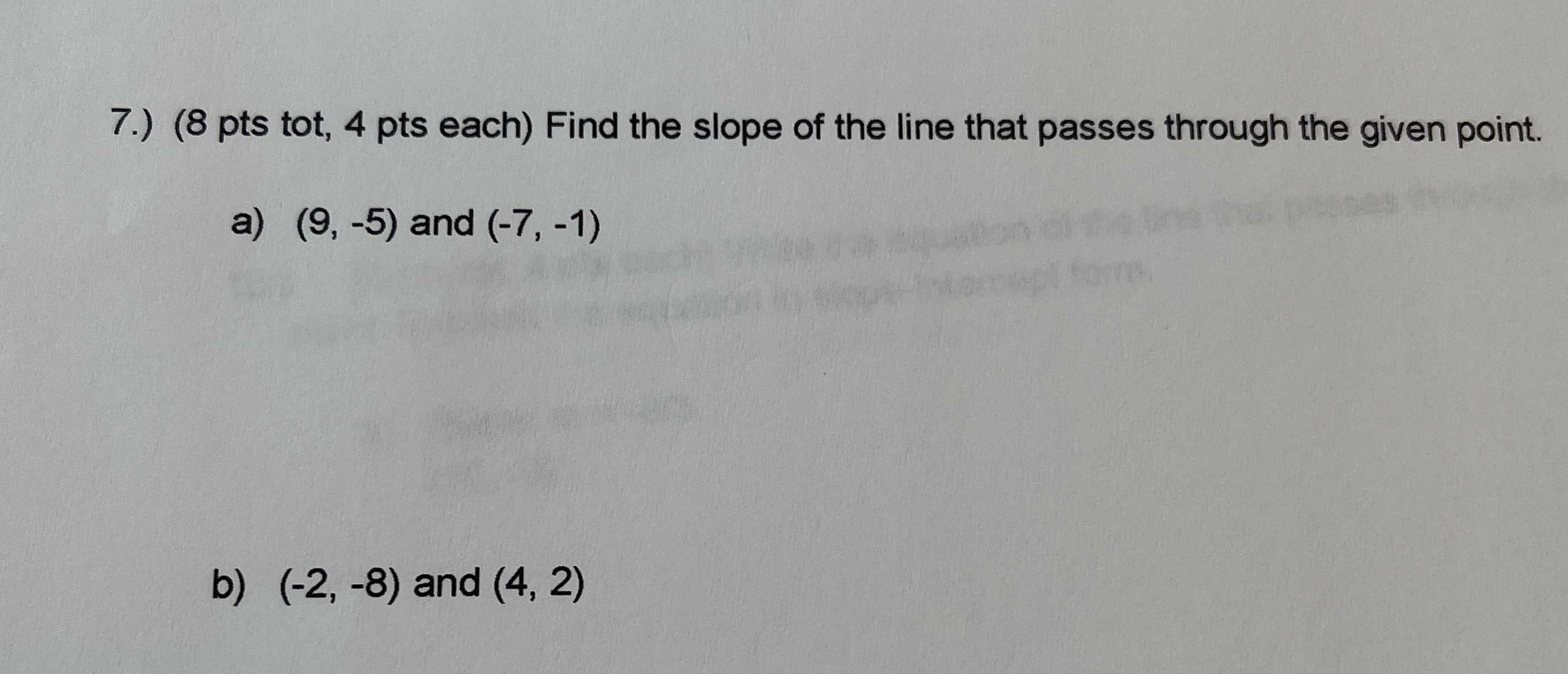### ¿Todavía tienes preguntas de matemáticas?

Pregunte a nuestros tutores expertos
Algebra
Pregunta7.) ( $$8$$ pts tot, $$4$$ pts each) Find the slope of the line that passes through the given point.

a) $$( 9 , - 5 )$$ and $$( - 7 , - 1 )$$

b) $$( - 2 , - 8 )$$ and $$( 4,2 )$$